# SCERT Maharashtra Question Bank solutions for 10th Standard SSC Science and Technology 1 Maharashtra State Board 2021 chapter 8 - Metallugy [Latest edition]

#### Chapters## Chapter 8: Metallugy

Choose the correct option.Find the correlation.Find odd one out.Match the columnsWrite the nameRight or Wrong sentenceIdentify who I am!Write scientific reasonsSolve the following QuestionsDistinguish betweenExplain the following reactions with the balanced equationsExplain concept with example/explain with the help of balanced equationDraw a neat labelled diagramComplete flow chart given belowAnswer the FollowingAnswer the following questions
Choose the correct option.

### SCERT Maharashtra Question Bank solutions for 10th Standard SSC Science and Technology 1 Maharashtra State Board 2021 Chapter 8 Metallugy Choose the correct option.

#### Choose the correct option.

Choose the correct option. | Q 1

Bronze is an alloy of ______.

• copper and tin

• copper and zinc

• tin and zinc

• copper, zinc and tin

Choose the correct option. | Q 2

_______ is an alloy made from iron, carbon and chromium.

• brass

• bronze

• stainless steel

• amalgam

Choose the correct option. | Q 3

_______ is basic oxide.

• CO2

• K2O

• SO2

• Al2O3

Choose the correct option. | Q 4

In electrolytic reduction of alumina _______ is used as a cathode.

• sulphur

• graphite

• platinum

• aluminium

Choose the correct option. | Q 5

Iron is _______.

• more reactive than zinc

• more reactive than aluminium

• less reactive than copper

• less reactive than aluminium

Choose the correct option. | Q 6

If Cu, Fe, Zn, Al elements are arranged in increasing order of their reactivity then the correct order would be which of the following?

• Cu, Fe, Zn, Al

• Al, Cu, Fe, Zn

• Zn, Al, Cu, Fe

• Fe, Zn, Al, Cu

Choose the correct option. | Q 7

Which of the following method is used to prevent the accumulation of greenish layer on brass due to corrosion?

• electroplating

• anodization

• tinning

• alloying

Choose the correct option. | Q 8

In Wilfley table method to separate particles of gangue _______ method is used.

• Magnetic

• Froth floatation

• Leaching

• gravitation

Choose the correct option. | Q 9

Aluminium oxide is _______ oxide.

• acidic

• basic

• neutral

• amphoteric

Choose the correct option. | Q 10

Atomic number of aluminium is _______ and its electronic configuration is _______.

• 13, (2, 8, 3)

• 12, (2, 8, 2)

• 13, (3, 10)

• 12, (2, 10)

Choose the correct option. | Q 11

The chemical formula of zinc blend is _______.

• ZnSO4

• ZnS

• ZnCO3

• ZnO

Choose the correct option. | Q 12

Extraction of moderately reactive elements is done by _______ and _______ method.

• roasting and calcination

• roasting and reduction

• separation and calcination

• none of these

Choose the correct option. | Q 13

Corrosion of silver causes a black layer of _______.

• Silver nitrate

• silver oxide

• silver sulphide

• silver carbonate

Choose the correct option. | Q 14

To prevent corrosion of iron and steel _______ method is used.

• electroplating

• anodization

• tinning

• galvanizing

Choose the correct option. | Q 15

In preparation of Aqua regia hydrochloric acid and _______ acid are mixed.

• sulphuric acid

• nitric acid

• carbonic acid

• phosphoric acid

Choose the correct option. | Q 16

The sound of one metal colliding with another makes a noise, this property is called as _______.

• good conductors

• ductility

• sonority

• malleability

Choose the correct option. | Q 17

Ionic compounds are electrically _______.

• positively charged

• negatively charged

• neutral

• conductor

Choose the correct option. | Q 18

_______ is good conductor of heat but bad conductor of electricity.

• graphite

• diamond

• coal

• iodine

Choose the correct option. | Q 19

_______ is the least reactive metal.

• silver

• sodium

• zinc

• gold

Choose the correct option. | Q 20

_______ forms a green colour in the water.

• CuSO4

• FeSO4

• NaCl

• all the above

Choose the correct option. | Q 21

Stainless steel is an alloy of _______.

• copper

• tin

• zinc

• iron

Choose the correct option. | Q 22

When one of the metals in an alloy is mercury the alloy is called _______.

• amalgam

• sodium amalgam

• zinc amalgam

• all the above

Choose the correct option. | Q 23

The minerals from which the metal can be separated economically are called _______.

• minerals

• ores

• gangue

• alloy

Find the correlation.

### SCERT Maharashtra Question Bank solutions for 10th Standard SSC Science and Technology 1 Maharashtra State Board 2021 Chapter 8 Metallugy Find the correlation.

#### Find the correlation.

Find the correlation. | Q 1

Brass : Aluminium and zinc : : Bronze : ______

Find the correlation. | Q 2

Pressure cooker : Anodizing : : Silver plated spoons : _______

Find the correlation. | Q 3

In Electrolytic reduction of alumina, Anode : _______ : : Cathode : Graphite lining

Find the correlation. | Q 4

Sulphide ores : Roasting : : Carbonate ores : _______

Find the correlation. | Q 5

Bauxite : Aluminium ore : : Cassiterite : _______

Find the correlation. | Q 6

Metal sheets : Malleable : : Electric wires : _______

Find the correlation. | Q 7

Zinc sulphide : Roasting : : zinc carbonate : _______

Find the correlation. | Q 8

Rusting of iron : Fe2O3 : : corrosion of copper : ______

Find the correlation. | Q 9

Diamond : electric insulator : : _______ : electric conductor

Find the correlation. | Q 10

Soft metal : Na : : hard metal : _______

Find the correlation. | Q 11

Aluminium: _______: gold : : electric insulator

Find the correlation. | Q 12

Bronze : _______ : : Stainless steel : Fe + Cr + C

Find odd one out.

### SCERT Maharashtra Question Bank solutions for 10th Standard SSC Science and Technology 1 Maharashtra State Board 2021 Chapter 8 Metallugy Find odd one out.

Find odd one out. | Q 1

Find the odd one out and give its explanation.

• Sodium

• Potassium

• Silver

• Sulphur

Find odd one out. | Q 2

Find the odd one out and give its explanation.

• Boron

• Chlorine

• Bromine

• Fluorine

Find odd one out. | Q 3

Find the odd one out and give its explanation.

• Copper

• Iron

• Mercury

• Brass

Find odd one out. | Q 4

Find the odd one out and give its explanation.

• Brass

• Bronze

• Phosphorous

• Steel

Find odd one out. | Q 5

Find the odd one out and give its explanation.

• Tinning

• Alloying

• Anodization

• Froth floatation

Find odd one out. | Q 6

Find the odd one out and give its explanation.

• Zinc coating

• Tinning

• Electroplating

• Calcination

Find odd one out. | Q 7

Find the odd one out and give its explanation.

• Na

• K

• Cu

• Li

Match the columns

### SCERT Maharashtra Question Bank solutions for 10th Standard SSC Science and Technology 1 Maharashtra State Board 2021 Chapter 8 Metallugy Match the columns

Match the columns | Q 1

Make pairs of substances and their properties

 Substance Property a. Potassium bromide (KBr) 1. Combustible b. Gold 2. Soluble in water c. Sulphur 3. No chemical reaction d. Neon 4. High ductility.
Match the columns | Q 2

Identify the pairs of metals and their ores from the following.

 Group A Group B a. Bauxite i. Mercury b. Cassiterite ii. Aluminium c. Cinnabar iii. Tin
Match the columns | Q 3

Match the columns.

 Group A Group B 1. ZnS a) Copper Sulphide 2. HgS b) Bauxite c) Cinnabar d) Zinc blend
Match the columns | Q 4

Match the columns.

 Group A Group B 1) Copper and Zinc a) Brass 2) Copper and Tin b) Bronze c) Stainless steel
Match the columns | Q 5

Match the columns.

 Group A Group B 1) Electroplating a) Pressure cooker 2) Anodising b) Silver plated spoons c) Coating of tin on copper d) Coating of Zinc on iron
Match the columns | Q 6

Match the columns.

 Group A Group B 1. Making sheets of metals a) Sonority 2. Making metal utensils b) Malleability 3. Making Copper wires c) Good conductor of heat 4. Making bells from metal d) Ductility
Write the name

### SCERT Maharashtra Question Bank solutions for 10th Standard SSC Science and Technology 1 Maharashtra State Board 2021 Chapter 8 Metallugy Write the name

Write the name | Q 1

Write the name.

The molecular formula of main ore of aluminium –

Write the name | Q 2

Write name.

The device used for grinding an ore.

Write the name | Q 3

Write the name.

Nonmetal which is a good conductors of electricity.

Write the name | Q 4

Write the name.

The reagent which dissolves noble metals.

Write the name | Q 5

Write the name.

Metals which are amphoteric in nature.

Write the name | Q 6

Write the name.

An alloy of copper and tin-

Write the name | Q 7

Write the name.

Two highly reactive metals-

Write the name | Q 8

Write the name.

The process of strong heating of carbonate ores in insufficient air–

Write the name | Q 9

Write the name.

The process of extraction of aluminium from alumina-

Write the name | Q 10

Write the name.

Method used to prevent corrosion of copper.

Right or Wrong sentence

### SCERT Maharashtra Question Bank solutions for 10th Standard SSC Science and Technology 1 Maharashtra State Board 2021 Chapter 8 Metallugy Right or Wrong sentence

Right or Wrong sentence | Q 1

Electrolysis is used to obtain pure metal from impure metal.

• Right

• Wrong

Right or Wrong sentence | Q 2

Ionic compounds are soluble in kerosene.

• Right

• Wrong

Right or Wrong sentence | Q 3

In solid state, ionic compounds conduct electricity.

• Right

• Wrong

Right or Wrong sentence | Q 4

Mercury, silver, gold are highly reactive metals.

• Right

• Wrong

Right or Wrong sentence | Q 5

In the electrolytic method, a layer of highly active metal is applied to a less active metal.

• Right

• Wrong

Right or Wrong sentence | Q 6

In the electrolytic reduction of alumina, the lining of graphite acts as an anode.

• Right

• Wrong

Right or Wrong sentence | Q 7

The electrolysis of alumina involves the use of fluorspar and cryolite to increase the melting point.

• Right

• Wrong

Right or Wrong sentence | Q 8

Cassiterite is a copper ore.

• Right

• Wrong

Right or Wrong sentence | Q 9

Diamond is a hard substance.

• Right

• Wrong

Right or Wrong sentence | Q 10

Gold and silver are active metals.

• Right

• Wrong

Right or Wrong sentence | Q 11

Halogen reacts with acid.

• Right

• Wrong

Right or Wrong sentence | Q 12

Bauxite reacts with sodium hydroxide in the Bayer’s process.

• Right

• Wrong

Identify who I am!

### SCERT Maharashtra Question Bank solutions for 10th Standard SSC Science and Technology 1 Maharashtra State Board 2021 Chapter 8 Metallugy Identify who I am!

Identify who I am! | Q 1

Identify who I am!

Carbon allotropes- _______

Identify who I am! | Q 2

Identify who I am!

Amphoteric oxide forming metal- _______

Identify who I am! | Q 3

Identify who I am!

Ore of Aluminum- ______

Identify who I am! | Q 4

Identify who I am!

Metal in Liquid state- _______

Write scientific reasons

### SCERT Maharashtra Question Bank solutions for 10th Standard SSC Science and Technology 1 Maharashtra State Board 2021 Chapter 8 Metallugy Write scientific reasons

Write scientific reasons | Q 1

Write scientific reason.

Sodium is always kept in kerosene.

Write scientific reasons | Q 2

Write scientific reason.

Pine oil is used in froth flotation.

Write scientific reasons | Q 3

Write scientific reason.

Lemon or tamarind is used for cleaning copper vessels turned greenish.

Write scientific reasons | Q 4

Write scientific reason.

Anodes need to be replaced from time to time during the electrolysis of alumina.

Write scientific reasons | Q 5

Write scientific reason.

Generally the ionic compounds have high melting points.

Write scientific reasons | Q 6

Write scientific reason.

Anodization method is useful for prevention of the corrosion of the aluminium.

Write scientific reasons | Q 7

Write scientific reason.

On exposure to air, silver articles turn blackish after some time.

Write scientific reasons | Q 8

Write scientific reason.

Magnetic separation method is used to separate the magnetic ingredients in the ores.

Write scientific reasons | Q 9

Write scientific reason.

Coins are made from metals and alloys.

Write scientific reasons | Q 10

Write scientific reason.

Meena’s mother uses lemon or tamarind for cleaning copper vessels turned greenish.

Write scientific reasons | Q 11

Write scientific reason.

Sodium is always kept in kerosene.

Solve the following Questions

### SCERT Maharashtra Question Bank solutions for 10th Standard SSC Science and Technology 1 Maharashtra State Board 2021 Chapter 8 Metallugy Solve the following Questions

Solve the following Questions | Q 1

When a copper coin is dipped in the solution of silver nitrate, it shows a lustre on its surface. Explain this process with the help of a chemical equation.

Solve the following Questions | Q 2

Electronic configuration of metal A is 2,8,1. Electronic configuration of metal B is 2,8,8,2. Which of the above metals is more reactive? Explain with reason.

Solve the following Questions | Q 3

Classify the following metals based on their reactivity.
Cu, Zn, Ca, Mg, Fe, Na, Li, Hg

 More reactive Moderately reactive Less reactive
Solve the following Questions | Q 4.1

Write the molecular formulae of the following compound.

Cryolite

Solve the following Questions | Q 4.2

Write the molecular formulae of the following compound.

Fluorspar

Solve the following Questions | Q 4.3

Write the molecular formulae of the following compound.

Sodium aluminate

Solve the following Questions | Q 4.4

Write the molecular formulae of the following compound.

Copper pyrite

Solve the following Questions | Q 4.5

Write the molecular formulae of the following compound.

Stannic oxide

Solve the following Questions | Q 4.6

Write the molecular formulae of the following compound.

Ferrous tungstate

Solve the following Questions | Q 5.1

Explain the concept of Roasting.

Solve the following Questions | Q 5.2

Explain the concept of Calcination.

Solve the following Questions | Q 6.1

What is an alloy?

Solve the following Questions | Q 6.2

Give two examples of alloy.

Solve the following Questions | Q 7

Explain Bayer’s process.

Solve the following Questions | Q 8

What is the difference between calcination and roasting?

Solve the following Questions | Q 9

Draw a neat and labelled diagram for Froth Floatation Process.

Solve the following Questions | Q 10

Explain the reactions of nonmetals with water with the help of examples.

Solve the following Questions | Q 11

Explain the characteristics of ionic compounds.

Solve the following Questions | Q 12

What are amphoteric oxides? Give two examples of amphoteric oxides.

Distinguish between

### SCERT Maharashtra Question Bank solutions for 10th Standard SSC Science and Technology 1 Maharashtra State Board 2021 Chapter 8 Metallugy Distinguish between

Distinguish between | Q 1

Distinguish between:

Metals - Nonmetals (physical characteristics)

Distinguish between | Q 2

Compare roasting and calcination.

Distinguish between | Q 3

Distinguish between:

Froth floatation - Leaching

Explain the following reactions with the balanced equations

### SCERT Maharashtra Question Bank solutions for 10th Standard SSC Science and Technology 1 Maharashtra State Board 2021 Chapter 8 Metallugy Explain the following reactions with the balanced equations

Explain the following reactions with the balanced equations | Q 1

Explain the following reaction with the balanced equation.

Sodium burns in air

Explain the following reactions with the balanced equations | Q 2

Explain the following reaction with the balanced equation.

Reaction of aluminium with oxygen

Explain the following reactions with the balanced equations | Q 3

Explain the following reaction with the balanced equation.

Magnesium reacts with dil HCl

Explain the following reactions with the balanced equations | Q 4

Explain the following reaction with the balanced equation.

Sulphur burns in air

Explain the following reactions with the balanced equations | Q 5

Explain the following reaction with the balanced equation.

Chlorine dissolved in water

Explain the following reactions with the balanced equations | Q 6

Explain the following reaction with the balanced equation.

Sodium aluminate reacts with water

Explain the following reactions with the balanced equations | Q 7

Write chemical equation for the event.

Iron filings are dropped in aqueous solution of copper sulphate.

Explain the following reactions with the balanced equations | Q 8

Explain the following reaction with the balanced equation.

Ferric oxide is reacted with aluminium.

Explain the following reactions with the balanced equations | Q 9

Write chemical equation for the event.

Electrolysis of alumina is done.

Explain the following reactions with the balanced equations | Q 10

Explain the following reaction with the balanced equation.

Dry aluminium hydroxide is ignited at 1000 °C

Explain the following reactions with the balanced equations | Q 11

Explain the following reaction with the balanced equation.

Zinc sulphide is heated strongly in excess of air

Explain the following reactions with the balanced equations | Q 12

Explain the following reaction with the balanced equation.

Zinc carbonate is heated strongly in limited supply of air

Explain the following reactions with the balanced equations | Q 13

Explain the following reaction with the balanced equation.

Zinc oxide is treated with carbon

Explain concept with example/explain with the help of balanced equation

### SCERT Maharashtra Question Bank solutions for 10th Standard SSC Science and Technology 1 Maharashtra State Board 2021 Chapter 8 Metallugy Explain concept with example/explain with the help of balanced equation

Explain concept with example/explain with the help of balanced equation | Q 1

Explain concept with example/explain with the help of a balanced equation.

Ionic bond and ionic compounds

Explain concept with example/explain with the help of balanced equation | Q 2

Explain concept with example/explain with the help of a balanced equation.

Gangue

Explain concept with example/explain with the help of balanced equation | Q 3

Explain concept with example/explain with the help of a balanced equation.

Ores

Explain concept with example/explain with the help of balanced equation | Q 4.1

Explain concept with example/explain with the help of a balanced equation.

Roasting

Explain concept with example/explain with the help of balanced equation | Q 4.2

Explain concept with example/explain with the help of a balanced equation.

Calcination

Explain concept with example/explain with the help of balanced equation | Q 5

Explain concept with example/explain with the help of a balanced equation.

Corrosion

Explain concept with example/explain with the help of balanced equation | Q 6

Explain concept with example/explain with the help of a balanced equation.

Minerals

Draw a neat labelled diagram

### SCERT Maharashtra Question Bank solutions for 10th Standard SSC Science and Technology 1 Maharashtra State Board 2021 Chapter 8 Metallugy Draw a neat labelled diagram

Draw a neat labelled diagram | Q 1

Draw a neat labelled diagram.

Magnetic separation method.

Draw a neat labelled diagram | Q 2

Draw a neat labelled diagram.

Froth floatation method.

Draw a neat labelled diagram | Q 3

Draw a neat labelled diagram.

Electrolytic reduction of alumina.

Draw a neat labelled diagram | Q 4

Draw a neat labelled diagram.

Hydraulic separation method.

Draw a neat labelled diagram | Q 5

Draw a neat labelled diagram.

Electroplating

Draw a neat labelled diagram | Q 6

Draw a neat labelled diagram.

Anodizing

Complete flow chart given below

### SCERT Maharashtra Question Bank solutions for 10th Standard SSC Science and Technology 1 Maharashtra State Board 2021 Chapter 8 Metallugy Complete flow chart given below

Complete flow chart given below | Q 1

Complete flow chart given below.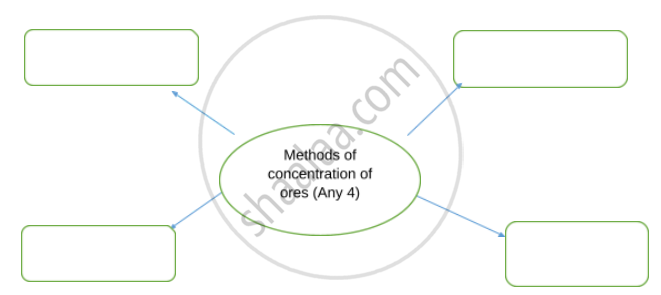Complete flow chart given below | Q 2

Complete flow chart given below.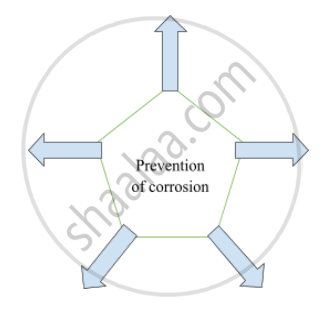Complete flow chart given below | Q 3

Complete flow chart given below.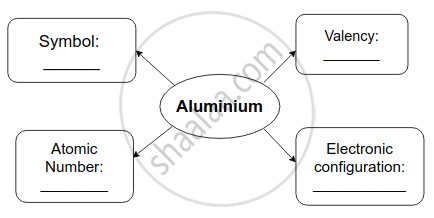### SCERT Maharashtra Question Bank solutions for 10th Standard SSC Science and Technology 1 Maharashtra State Board 2021 Chapter 8 Metallugy Answer the Following

Answer the Following | Q 1

What is corrosion?

Answer the Following | Q 2

What is rust?

Answer the Following | Q 3

Write a molecular formula for rust.

Answer the Following | Q 4

Observe the following diagram and identify the type of reaction and write observation.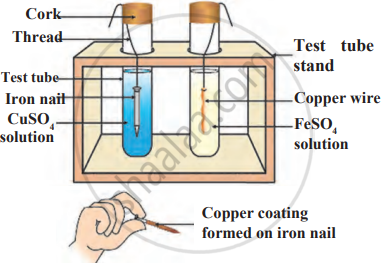Answer the Following | Q 5

Observe the following diagram and give answers.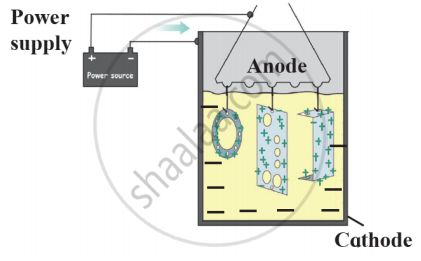1. Name this method of prevention of corrosion.
2. For prevention of which metal this method is used?
3. What is used as anode in this method?
Answer the Following | Q 6

Explain the hydraulic separation method with a neat labelled diagram.

Answer the Following | Q 7

Observe the following diagram and write answers.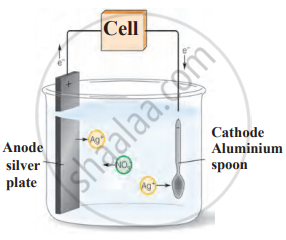1. Name the method.
2. Explain the method.
3. Give two examples of this method.
Answer the Following | Q 8

Observe the following diagram and write answers.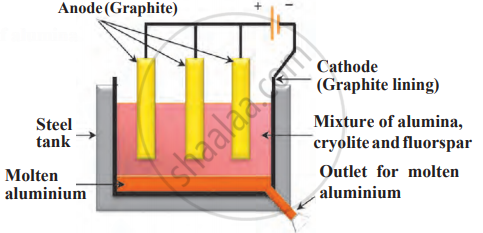1. Name the method.
2. Write anode reaction and cathode reaction.
3. Why fluorspar and cryolite are added in the mixture?
Answer the Following | Q 9

Identify the following method of concentration of ores and explain briefly.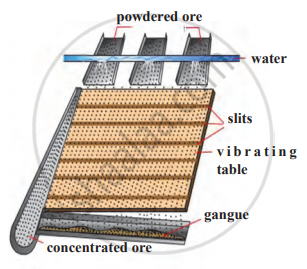Answer the Following | Q 10

Observe the given figure of reactivity series of metals and answer the following questions: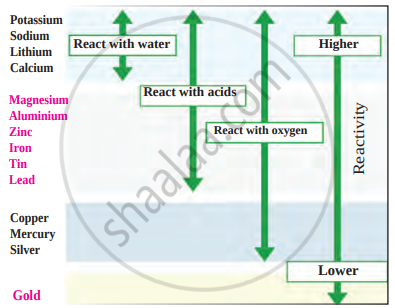Reactivity series of metals

1. Name two metals which react with water.
2. Name two moderately reactive metals.
3. Name the most highly reactive metal and the most less reactive metal.
Answer the Following | Q 11

Explain the froth floatation method with a neat labelled diagram.

Answer the Following | Q 12

According to the reactivity series, zinc is more reactive than iron, iron is more reactive than silver. During study of this, a student dipped the iron nails in silver nitrate solution.

1. What is reactivity series?
2. What will happen when iron nails are dipped in silver nitrate solution?
3. Which type of reaction happens when iron metal reacts with silver nitrate solution?
4. What will happen if a zinc rod is used instead of iron nail?
Answer the Following | Q 13

Complete the following flowchart.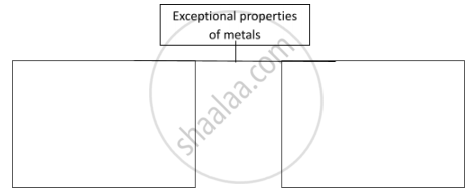Answer the Following | Q 14

Complete the following flowchart.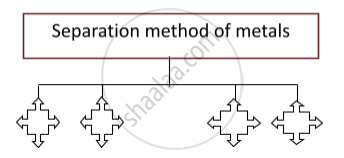Answer the Following | Q 15

Complete the following flow chart.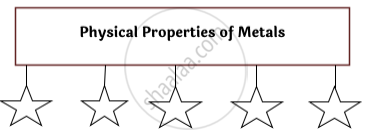Answer the Following | Q 16

Write the properties of ionic compounds.

### SCERT Maharashtra Question Bank solutions for 10th Standard SSC Science and Technology 1 Maharashtra State Board 2021 Chapter 8 Metallugy Answer the following questions

Answer the following questions | Q 1.1

Explain in brief types of extraction of highly reactive metals according to their reactivity.

Answer the following questions | Q 1.2

Explain in brief types of extraction of moderately reactive metals according to their reactivity.

Answer the following questions | Q 1.3

Explain in brief types of extraction of less reactive metals according to their reactivity.

Answer the following questions | Q 2

Explain Bayer’s process of concentration of bauxite with a chemical equation.

Answer the following questions | Q 3

Explain in brief electrolytic reduction of alumina with a neat labelled diagram.

Answer the following questions | Q 4

Observe the figure and answer the following.1. Write the name of the method.
2. What is used as anode and cathode in this method?
3. Write the molecular formula and use of cryolite.
4. Write anode reaction.
5. Write cathode reaction.
Answer the following questions | Q 5.1

Observe the figure and name and explain in brief the following method: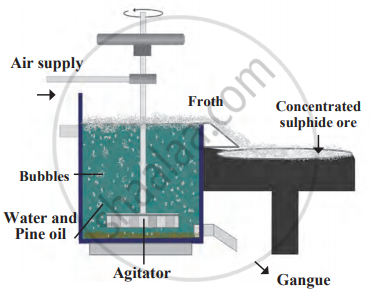Answer the following questions | Q 5.2

Observe the figure and name and explain in brief the following method: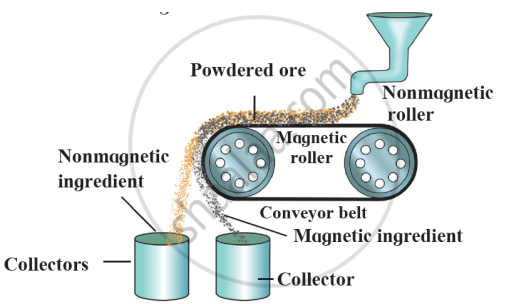Answer the following questions | Q 6

Answer the questions on the following passage.

The minerals from which the metal can be separated economically are called ores. Ores contain many types of impurities such as soil, sand and rocky substances along with metal compounds. These impurities are called gangue.
Metals can be extracted from their ores by means of various methods of separation. The process of extraction of metal in a pure state from the ores is also a part of metallurgy.
Ores are taken out from the mines and the gangue is usually separated from the ore at the site itself by various methods. Then the ores are carried out to the place where metals are produced. Here metals are extracted in pure form. Then metals are further purified by different methods of purification. This entire process is called metallurgy. Most metals being reactive do not occur in nature in the free state but are found in combined state as their salts such as oxides, carbonates, sulphides, and nitrates. however, the most unreactive metals that are not affected by air, water and other natural factors like silver, gold, platinum, generally occur in a free state. The compounds of metals that occur in nature along with the impurities are called minerals.

1. What are ores?
2. Which processes are involved in the branch of metallurgy? What is metallurgy?
3. Which metals are found in a free state?
4. In what forms are metals found in combined state?
5. What is gangue?
Answer the following questions | Q 7

Explain the difference between Bayer’s process and Hall’s process by explaining the Bayer’s process.

Answer the following questions | Q 8.1

What is corrosion?

Answer the following questions | Q 8.2

Give preventive methods by giving examples of corrosion?

## Chapter 8: Metallugy

Choose the correct option.Find the correlation.Find odd one out.Match the columnsWrite the nameRight or Wrong sentenceIdentify who I am!Write scientific reasonsSolve the following QuestionsDistinguish betweenExplain the following reactions with the balanced equationsExplain concept with example/explain with the help of balanced equationDraw a neat labelled diagramComplete flow chart given belowAnswer the FollowingAnswer the following questions## SCERT Maharashtra Question Bank solutions for 10th Standard SSC Science and Technology 1 Maharashtra State Board 2021 chapter 8 - Metallugy

SCERT Maharashtra Question Bank solutions for 10th Standard SSC Science and Technology 1 Maharashtra State Board 2021 chapter 8 (Metallugy) include all questions with solution and detail explanation. This will clear students doubts about any question and improve application skills while preparing for board exams. The detailed, step-by-step solutions will help you understand the concepts better and clear your confusions, if any. Shaalaa.com has the Maharashtra State Board 10th Standard SSC Science and Technology 1 Maharashtra State Board 2021 solutions in a manner that help students grasp basic concepts better and faster.

Further, we at Shaalaa.com provide such solutions so that students can prepare for written exams. SCERT Maharashtra Question Bank textbook solutions can be a core help for self-study and acts as a perfect self-help guidance for students.

Concepts covered in 10th Standard SSC Science and Technology 1 Maharashtra State Board 2021 chapter 8 Metallugy are Types of Elements: Metals, Types of Elements: Non-metal, Corrosion of Metals and Its Prevention, Types of Separation or Concentration of an Ore, Physical Properties of Metals, Electrovalent (or Ionic) Bond, Extraction of Aluminium, Chemical Properties of Metal, Physical Properties of Non-metal, Chemical Properties of Non-metal, Reactivity Series of Metals, Ionic Compounds and Electrical Conductivity, Metallurgy, Extraction of Metals, Refining of Metals.

Using SCERT Maharashtra Question Bank 10th Standard [इयत्ता १० वी] solutions Metallugy exercise by students are an easy way to prepare for the exams, as they involve solutions arranged chapter-wise also page wise. The questions involved in SCERT Maharashtra Question Bank Solutions are important questions that can be asked in the final exam. Maximum students of Maharashtra State Board 10th Standard [इयत्ता १० वी] prefer SCERT Maharashtra Question Bank Textbook Solutions to score more in exam.

Get the free view of chapter 8 Metallugy 10th Standard [इयत्ता १० वी] extra questions for 10th Standard SSC Science and Technology 1 Maharashtra State Board 2021 and can use Shaalaa.com to keep it handy for your exam preparation